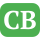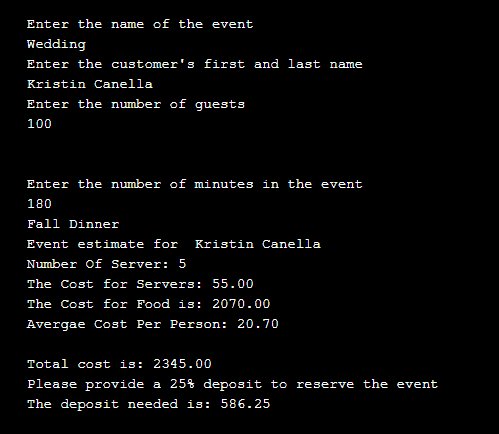CppBuzz.com

# C++ Project on Catering with Source Code

Basic project to get estimates of Catering cost

## Source Code

```/*
Author: https://www.cppbuzz.com
Date: 9th Feb 2019
Compiled on: g++
1. Enter the Name of Event
2. Enter number of guests
3. Enter duration of events

For more projects please visit : https://www.cppbuzz.com
If you get any compilation issue then write email to admin@cppbuzz.com
*/

//You can give any name for this file since it is standalone file.

#include <iostream>
#include <string>
#include <iomanip>
#include <cmath>

using namespace std;

int main()
{
string NameOfEvent, CustomerName;	// Name of Event + Customer Name
double NumberOfGuests;	// Number of Guests in attendance
int NumberOfMinutes;	// Number of Minutes in the event

// Information input (Events name and amounts)

cout << "Enter the name of the event " << endl;
getline(cin, NameOfEvent);
cout << "Enter the customer's first and last name " << endl;
getline(cin, CustomerName);
cout << "Enter the number of guests " << endl;
cin >> NumberOfGuests;
cout << "\n\nEnter the number of minutes in the event " << endl;
cin >> NumberOfMinutes;

cout << "Fall Dinner     " << endl;
cout << "Event estimate for  " << CustomerName << endl;

int NumberOfServers, Cost1;
double CostForOneServer,Test, TotalFoodCost, AverageCost, Cost2, DepositAmount,TotalCost;

const double CostPerHour = 18.50;
const double CostPerMinute = .40;
const double CostOfDinner = 20.70;

// Get Number Of Servers
Test = NumberOfGuests / 20;
NumberOfServers = ceil(Test);

// Get Cost Of One Server
Cost1 = (NumberOfMinutes / 60) * CostPerHour;
Cost2 = (NumberOfMinutes % 60) * CostPerMinute;
CostForOneServer = Cost1 + Cost2;

// Get Cost For Food
TotalFoodCost = NumberOfGuests * CostOfDinner;

// Get Average Cost Per Person
AverageCost = TotalFoodCost / NumberOfGuests;

// Get Total Cost
TotalCost = TotalFoodCost + (CostForOneServer * NumberOfServers);

// Get Deposit Amount (25%)
DepositAmount = TotalCost * .25;

// Print values above
cout << "Number Of Server: " << NumberOfServers << fixed << setprecision(2) << endl;
cout << "The Cost for Servers: " << setw(5) << CostForOneServer << endl;
cout << "The Cost for Food is: " << setw(5) << TotalFoodCost << endl;
cout << "Avergae Cost Per Person: " << setw(5) << AverageCost << endl;
cout << "\nTotal cost is: " << setw(5) << TotalCost << endl;

cout << "Please deposite a 25% deposit to reserve the event" << endl;
cout << "The deposit needed is: " << setw(5) << DepositAmount << endl;

return 0;
}
```

## Execution of Catering Project